### Home > CALC > Chapter 2 > Lesson 2.2.1 > Problem2-50

2-50.
1. Consider the functions f(x) = log(3 − x) and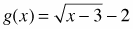. Homework Help ✎

1. What is the domain of each function?

2. Graph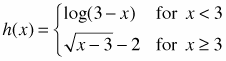on your graphing calculator.

3. Explain why h(x) is not continuous at x = 3?

4. What is the range of each function?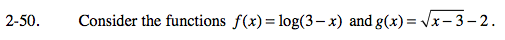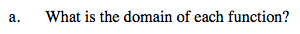Recall that domains of the parent functions:

The domain will shift with the function.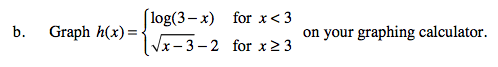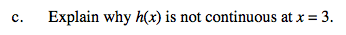Consider: x → 3, y → _________ and x → 3+, y → ________
Do the left and the right approach the same value?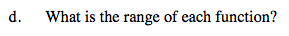Consider the range of each piece of the piecewise function separately.

Use the eTool below to view the graphs for part (b).
Click on the link to the right to view the full version of the eTool. Calc 2-50 HW eTool